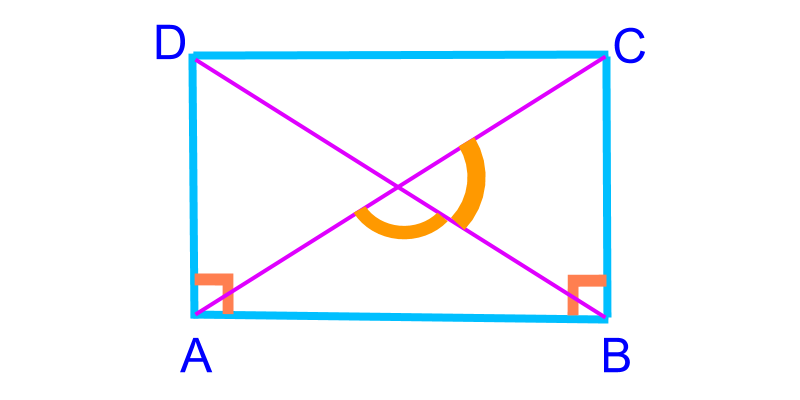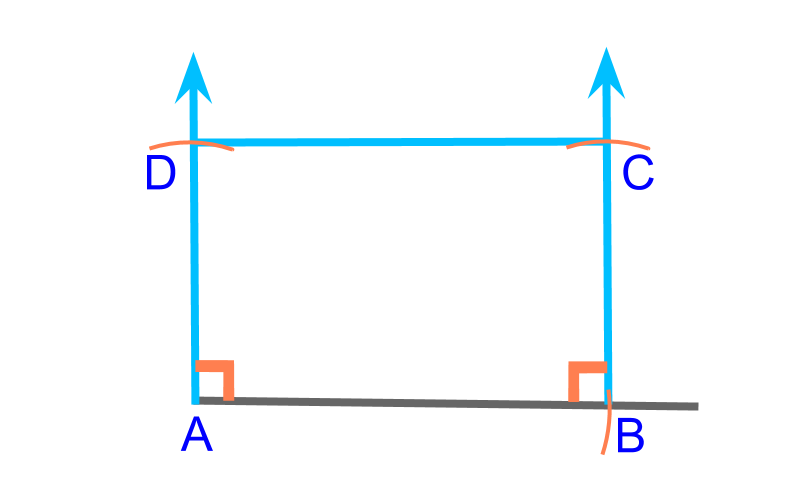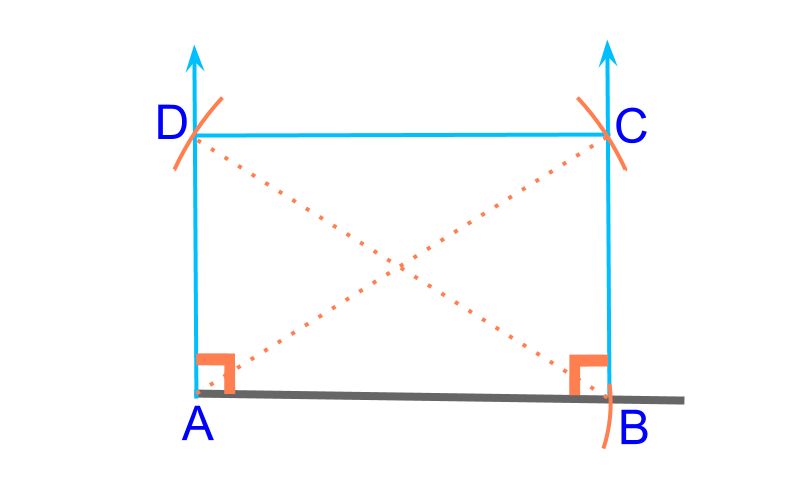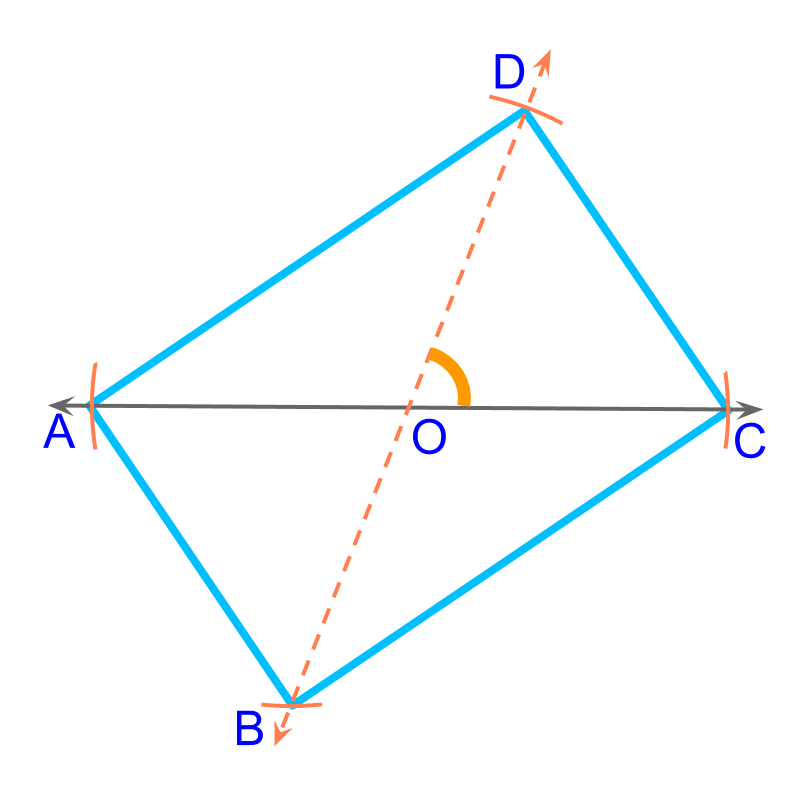maths > construction-basics

Construction of Rectangles

what you'll learn...

overview

In this page, constructing rectangles is explained. It is outlined as follows.

•  Properties of rectangles is explained

•  The number of independent parameters in a rectangle is 1$1$

•  For a given parameter, construction of rectangles is approached as combination of triangles (sss, sas, asa, rhs, sal) and using the properties of rectangles.

understanding rectangle

A rectangle is a parallelogram with all interior angles 90${90}^{\circ}$A quadrilateral is defined by 5$5$ parameters. A Parallelogram is defined by 3$3$ parameters. And for a rectangle, the following properties provide additional dependency of parameters.

•  all interior angles are 90${90}^{\circ}$

•  diagonals are equal and bisect

•  opposite sides are parallel and equal

•  two angles on diagonals are supplementary.

These properties cause one parameter to be dependent on other parameters and so, a rectangle is defined by 2$2$ parameters.To construct a rectangle, $2$ sides ($\overline{A B}$, $\overline{B C}$) are given. This is illustrated in the figure. To construct, consider this as two SAS triangles $A B C$ and $A B D$.To construct a rectangle, a side ($\overline{A B}$) and the diagonal ($\overline{A C}$) are given. This is illustrated in the figure. To construct, consider this as two RHS triangles $A B C$ and $A B D$.To construct a rectangle, the diagonal ($\overline{A C}$) and the angle between them ($\angle D O C$) are given. This is illustrated in the figure.

To construct the specified rectangle, "Consider this as two SAS triangle $C O D$ and $A O B$". Note: Use the property that diagonals bisect and mark the vertices at half diagonals.

summaryConstruction of Rectangles :

Properties of rectangle

•  all interior angles are ${90}^{\circ}$

•  diagonals are equal and bisect

•  opposite sides are parallel and equal

The formulations of questions

•  $2$ sides

•  $1$ side and the diagonal

•  the diagonal and an angle between the diagonals

use properties to figure out dependent parameters and look for triangles

Outline